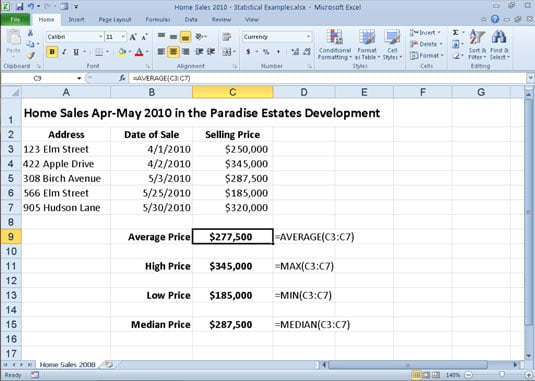##### Excel 2010 All-in-One For DummiesExcel 2010's Statistical functions — including AVERAGE, MAX, MIN, and MEDIAN — are found on a continuation menu accessed from the More Functions command button's drop-down menu on the Formulas tab. Excel includes one of the most complete sets of statistical functions available outside a dedicated statistics software program.

The AVERAGE, MAX (for maximum), and MIN (for minimum) functions are the most commonly used of the statistical functions because they are of use to both the average number cruncher as well as the dedicated statistician. All three functions follow the same syntax as the good old SUM function. For example, the syntax of the AVERAGE function uses the following arguments just as the SUM, MAX, and MIN functions do:

`=AVERAGE(number1,[<i>number2</i>],[...])`

Just as in the SUM function, you can use up to 30 number arguments for which you want the average. The figure below illustrates how you can use the AVERAGE, MAX, MIN, and MEDIAN functions in a worksheet. This example uses these functions to compute a few statistics on the selling prices of homes in a particular neighborhood. These statistics include the average, highest, lowest, and median selling price for the homes sold in April and May of 2010. All the statistical functions in this worksheet use the same number argument; that is, the cell range C3:C7. The formulas used in this worksheet are shown in column D.A home sales worksheet that uses common statistical functions.

The AVERAGE function computes the arithmetic mean of the values in this range by summing them and then dividing them by the number of values in the range. This AVERAGE function is equivalent to the following formula:

`=SUM(C3:C7)/COUNT(C3:C7)`

Note that this formula uses the SUM function to total the values and another statistical function called COUNT to determine the number of values in the list. The MAX and MIN functions simply compute the highest and lowest values in the cell range used as the number argument. The MEDIAN function computes the value that is in the middle of the range of values; that is, the one where half the values are greater and half are less. This is the reason that the median sales price (in cell C15) differs from the average sales price (in cell C9) in this worksheet.